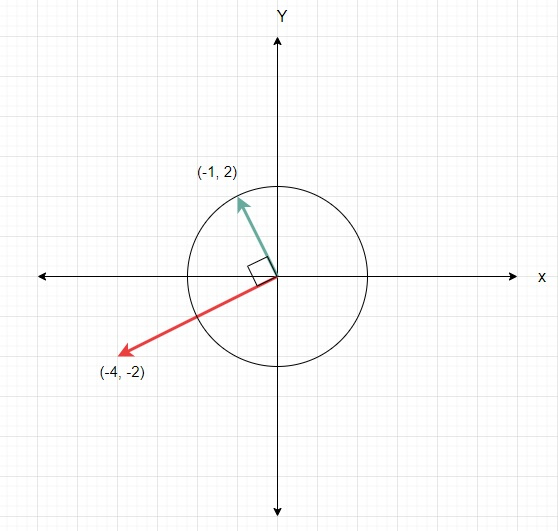# Check if it is possible to reach vector B by rotating vector A and adding vector C to its in Python

Suppose we have three vectors x, y and z in 2D plane. We have to check whether we can get vector y from vector x by rotating it 90 degrees(clockwise) or adding z with it any number of times as required.

So, if the input is like x = (-4, -2) y = (-1, 2) z = (-2, -1), then the output will be True as we can add z with x to get location (-2, -1), then rotate 90° clockwise to get (-1, 2).To solve this, we will follow these steps −

Define a function util() . This will take p, q, r, s

• d := r * r + s * s
• if d is same as 0, then
• return true when p and q both are 0, otherwise false
• return true when (p * r + q * s) and (q * r - p * s) both are divisible by d, otherwise false
• From the main method do the following −
• if any one of util(x of p - x of q, y of p - y of q, x of r, y of r) or util(x of p + x of q, y of p + q, x of r, y of r) or util(x of p - y of q, y of p + x of q, x of r, y of r) or util(x of p + y of q, y of p - x of q, x of r, y of r) is true, then
• return True
• return False

## Example

Let us see the following implementation to get better understanding −

Live Demo

def util(p, q, r, s):
d = r * r + s * s
if d == 0:
return p == 0 and q == 0
return (p * r + q * s) % d == 0 and (q * r - p * s) % d == 0
def solve(p,q,r):
if util(p - q, p - q, r, r) or util(p + q, p + q, r, r) or util(p - q, p + q, r, r) or util(p + q, p - q, r, r):
return True
return False
p = (-4, -2)
q = (-1, 2)
r = (-2, -1)
print(solve(p, q, r))

## Input

(-4, -2), (-1, 2), (-2, -1)

## Output

True